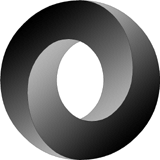22 August 2018### 如何将JSON中的数字和字符串都解析为数字？

``````var raw = []byte(`{"a":1}`)
var raw_string = []byte(`{"a":"2"}`)
``````

``````type S3 struct {
A json.Number `json:"a"`
}

s3 := new(S3)
err = json.Unmarshal(raw, s3)
log.Println(err, s3.A)

s31 := new(S3)
err = json.Unmarshal(raw_string, s31)
log.Println(err, s31.A)
``````

### 原理

``````// A Number represents a JSON number literal.
type Number string

// String returns the literal text of the number.
func (n Number) String() string { return string(n) }

// Float64 returns the number as a float64.
func (n Number) Float64() (float64, error) {
return strconv.ParseFloat(string(n), 64)
}

// Int64 returns the number as an int64.
func (n Number) Int64() (int64, error) {
return strconv.ParseInt(string(n), 10, 64)
}
``````

### UseNumber

``````decoder := json.NewDecoder(bytes.NewBufferString(`{"10000000000":10000000000,"111":1}`))
decoder.UseNumber()
var obj map[string]interface{}
decoder1.Decode(&obj)
``````

EOF

#### 欢迎加微信交流与吐槽